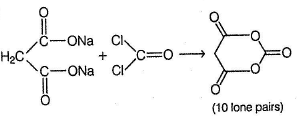Courses

# CPU 3 - Introduction To Acid Derivatives (Carboxylic Acids And Acid Derivatives)

## 21 Questions MCQ Test | CPU 3 - Introduction To Acid Derivatives (Carboxylic Acids And Acid Derivatives)

Description
This mock test of CPU 3 - Introduction To Acid Derivatives (Carboxylic Acids And Acid Derivatives) for Class 12 helps you for every Class 12 entrance exam. This contains 21 Multiple Choice Questions for Class 12 CPU 3 - Introduction To Acid Derivatives (Carboxylic Acids And Acid Derivatives) (mcq) to study with solutions a complete question bank. The solved questions answers in this CPU 3 - Introduction To Acid Derivatives (Carboxylic Acids And Acid Derivatives) quiz give you a good mix of easy questions and tough questions. Class 12 students definitely take this CPU 3 - Introduction To Acid Derivatives (Carboxylic Acids And Acid Derivatives) exercise for a better result in the exam. You can find other CPU 3 - Introduction To Acid Derivatives (Carboxylic Acids And Acid Derivatives) extra questions, long questions & short questions for Class 12 on EduRev as well by searching above.
QUESTION: 1

### Only One Option Correct Type Direction (Q. Nos. 1-6) This section contains 6 multiple choice questions. Each question has four choices (a), (b), (c) and (d), out of which ONLY ONE is correct. Q.  Which of the following is most easily decarboxylated on heating?

Solution:

β-keto undergo easier decarboxylation via six membered cyclic intermediate.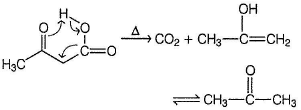QUESTION: 2

### Which of the following reaction fail to occur spontaneously as written?

Solution:

Here the more stable acid derivative ester is being converted into less stable anhydride.

QUESTION: 3

### Which of the following gives a cyclic anhydride on heating ?

Solution:

The two —COOH groups are syn, can form cyclic anhydride.

QUESTION: 4

What is the increasing order of reactivity of the followings towards base catalysed hydrolysis reaction?

I. CH3CONH2
II. CH3COOCH3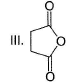IV. C6H5COCl

Solution:

Rate of hydrolysis follow the order of reactivity of acid derivatives towards nucleophilic acyl substitution.

QUESTION: 5

In the reaction given below, the major organic product formed is

Solution: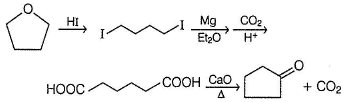QUESTION: 6

In the reaction given below, the major organic product is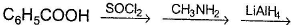Solution: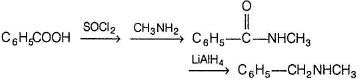*Multiple options can be correct
QUESTION: 7

One or More than One Options Correct Type

Direction (Q. Nos. 7-10) This section contains 4 multiple choice questions. Each question has four choices (a), (b), (c) and (d), out of which ONE or MORE THAN ONE are correct.

Q.

Which reagent(s) can convert benzoic acid into corresponding acid chloride?

Solution:

All these reagents convert acid into acid chloride. HCI does not react with RCOOH to give RCOCI.

*Multiple options can be correct
QUESTION: 8

Which reaction condition can convert butanoic acid into methyl butanoate?

Solution: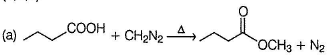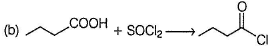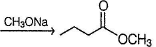(c) Amide do not undergo nucleophilic acyl substitution to give less stable acid derivative ester.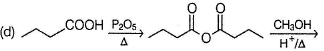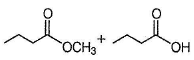*Multiple options can be correct
QUESTION: 9

What is/are correct regarding the following acyl substitution reaction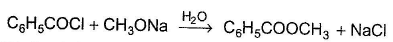Solution:

Nucleophilic acyl substitution shown above is bimolecular, second order reaction. Therefore, presence of electron withdrawing groups like —NO2 in the ring increases reactivity. Polar aprotic solvents like DMSO increases reactivity of bimolecular nucleophilic substitution reaction.

*Multiple options can be correct
QUESTION: 10

Which is/are not a suitable nucleophilic substitution reaction ?

Solution:

(a) Grignard reagent attack further on ketone. (c) Grignard reagent takes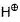from amide.

QUESTION: 11

Comprehension Type

Direction (Q. Nos. 11-13) This section contains a paragraph, describing theory, experiments, data, etc.
Three questions related to the paragraph have been given. Each question has only one correct answer among the four given options (a), (bj, (c) and (d).

Passage

In a nucleophilic acyl substitution reaction, a more reactive acid derivative is spontaneously converted into a more stable acid derivative :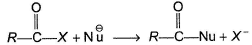Here X- could be Cl-, Br- , RO-, RCOO- or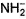. The reaction is found to be bimolecular.

Q.

The leaving ability of X- is in order of

Solution:

Stronger bases are stronger nucle ophiles (usually), hence poor leaving groups.

QUESTION: 12

In a nucleophilic acyl substitution reaction, a more reactive acid derivative is spontaneously converted into a more stable acid derivative :Here X- could be Cl-, Br- , RO-, RCOO- or. The reaction is found to be bimolecular.

Q.

If X is Cl, the increasing order of reactivity of the following with CH3ONa is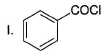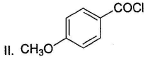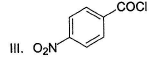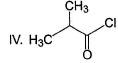Solution:

Electron withdrawing group on phenyl ring increases electrophilicity of carboxyl group and vice versa. Hence, III is most reactive and II is most stabl

QUESTION: 13

In a nucleophilic acyl substitution reaction, a more reactive acid derivative is spontaneously converted into a more stable acid derivative :Here X- could be Cl-, Br- , RO-, RCOO- or. The reaction is found to be bimolecular.

Q.

How benzoic acid X be most efficiently be converted into 2-phenyl propene?

Solution: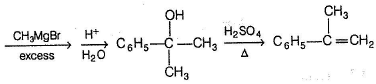QUESTION: 14

Statement Type

Direction (Q. Nos. 14-17) This section is based on Statement I and Statement II. Select the correct answer from the codes given below.

Q.

Statement I : Ethanoic anhydride on heating with ammonia gives ethanamide.

Statement II : Amide is more stable acid derivative than anhydride.

Solution:

In nucleophilic acyl substitution, a more reactive acid derivative is converted into a more stable acid derivative.

QUESTION: 15

Statement I : CH3COOH when treated with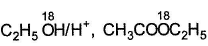is formed.

Statement II : In Fischer esterification, protonated acid undergo nucleophilic attack by alcohol in the slow, rate determining step.

Solution: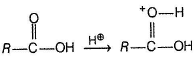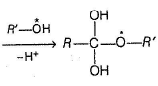The above intermediate dehydrate to give ester in which oxygen (starred) of alcohol is retained in ester.

QUESTION: 16

Statement I : 1-butene, when treated with acetic acid in the presence of H2SO4, produces racemic mixture of 2-butylpropanoate.

Statement II : Acetic acid undergo electrophilic addition at olefinic bond in the presence of an acid catalyst.

Solution:

Both are independently correct but formation of racemic mixture of ester is due to attack of acid on planar carbocation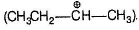QUESTION: 17

Statement I : In the following reaction,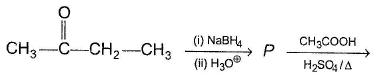Racemic mixture of esters.

Statement II : Alcohol (P) undergo nucleophilic addition on protonated acetic acid.

Solution:

Both are independently correct but formation of racemic mixture of esters is due to the formation of racemic mixture of alcohols (P) by hydride ion attack on planar carbonyl carbon of butanone.

*Answer can only contain numeric values
QUESTION: 18

One Integer Value Correct Type

Direction (Q. Nos. 18-21) This section contains 4 questions. When worked out will result in an integer from 0 to 9 (both inclusive).

Q.

A polycarboxylic acid (X) has neutralisation equivalent of 65.5. X when treated with excess of SOCI2 followed by excess of CH3ONa(aq), gives ester of molar mass 318. What is the basicity of original acid?

Solution:

(4) In the given reaction :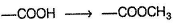A net gain in molar mass of 14 unit is observed per —COOH present. Let there be “n" —COOH group (basicity) be present.
Hence, molar mass of acid = 65.5 n
Molar mass of ester = 65.5n + 14n = 79.5n = 318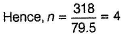*Answer can only contain numeric values
QUESTION: 19

Dicarboxylic acid HOOC —(CH2)n —COOH on heating alone undergo cyclisation giving cyclic anhydride. For this reaction, what should be the minimum values of n?

Solution:

If n is less than 2, decarboxylation is preferred over cyclisation.

*Answer can only contain numeric values
QUESTION: 20

If one of the isomer of a hydrocarbon on treatment with hot, concentrated alkaline, KMnO4 followed by acidic work up gives phthalic acid without loss of any carbon atoms, how many total isomers of hydrocarbon are possible, each containing a phenyl ring?

Solution: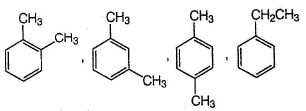*Answer can only contain numeric values
QUESTION: 21

If disodium salt of propanedioic acid is treated with excess of COCI2 a cyclic compound X is formed. How many lone pair of electrons are present in X?

Solution: